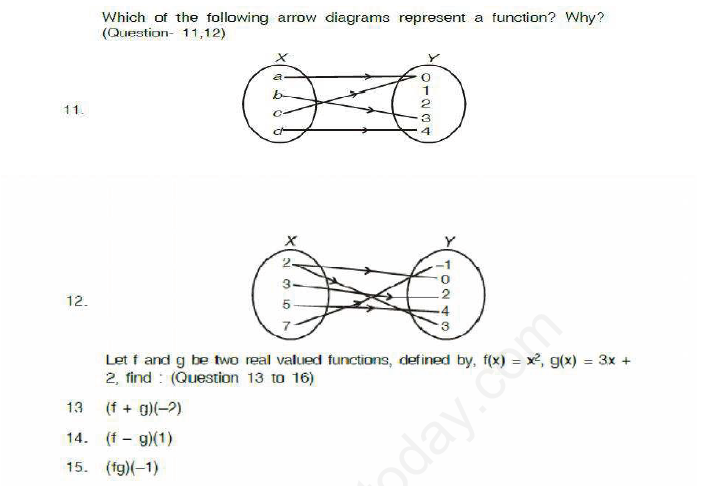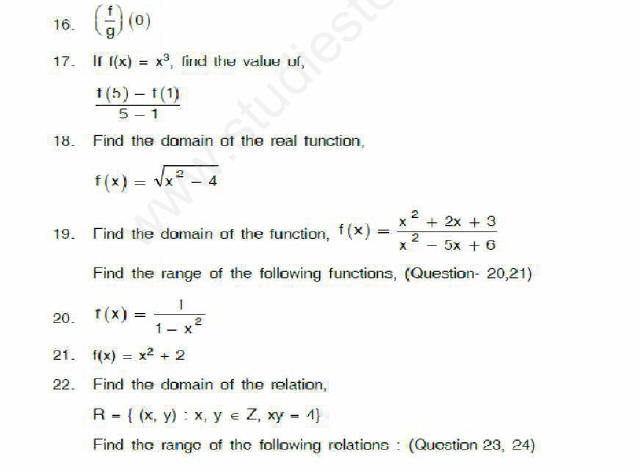# CBSE Class 11 Mathematics Sets Assignment Set A

Read and download free pdf of CBSE Class 11 Mathematics Sets Assignment Set A. Get printable school Assignments for Class 11 Mathematics. Standard 11 students should practise questions and answers given here for Chapter 1 Sets Mathematics in Grade 11 which will help them to strengthen their understanding of all important topics. Students should also download free pdf of Printable Worksheets for Class 11 Mathematics prepared as per the latest books and syllabus issued by NCERT, CBSE, KVS and do problems daily to score better marks in tests and examinations

## Assignment for Class 11 Mathematics Chapter 1 Sets

Class 11 Mathematics students should refer to the following printable assignment in Pdf for Chapter 1 Sets in standard 11. This test paper with questions and answers for Grade 11 Mathematics will be very useful for exams and help you to score good marks

### Sets MCQ Questions with Answers Class 11 Mathematics

Question : The number of elements in the Power set P(S) of the set S = [ [ Φ] , 1, [ 2, 3 ]] is
A. 4
B. 8
C. 2
D. None of these
Ans-B

Question :  If A and B are sets and A∪ B= A ∩ B, then
A. A= Φ
B. B= Φ
C. A=B
D. None of these
Ans-C

Question :   Let S be an infinite set and S1, S2, S3, ..., Sn be sets such that S1 ∪S2 ∪ S3∪....Sn = S then
A. atleast one of the sets Si is a finite set
B. not more than one of the set Si can be infinite
C. atleast one of the sets Si is an infinite set
D. none of these
Ans-C

Question :  If A be a finite set of size n, then number of elements in the power set of A x A
A. 22n
B. 2
C. (2n)2
D. none of these
Ans-B

Question :  Total number of different partitions of a set having four elements is
A. 16
B. 8
C. 15
D. 4
Ans-C

Question :  A - (B ∪ C) is
A. (A - B) ∪ (A - C)
B. A - B - C
C. (A - B) ∩ (A - C)
D. A - (B ∩ C)'
Ans-C

Question :  Which of the following sets are null sets?
A. {0}
B. Ø
C. { }
D. Both (b) & (c)
Ans-D

Question :  Number of subsets of a set of order three is
A. 3
B. 6
C. 8
D. 9
Ans-C

Question :  The number of elements in the power set of the set {{a, b}, c} is
A. 8
B. 4
C. 3
D. 7
Ans-C

Question :  In a language survey of students it is found that 80 students know English, 60 know French, 50 know German, 30 known English and French, 20 know French and German, 15 know English and German and 10 students know all the three languages. How many students know at least one language?
A. 135
B. 30
C. 10
D. 45
Ans-A

Question :  In a room containing 28 people, there are 18 people who speak English, 15 people who speak Hindi and 22 people who speak Kannada, 9 persons speak both English and Hindi, 11 persons speak both Hindi and Kannada where as 13 person speak  both Kannada and English. How+ many people speak all the three languages?
A. 6
B. 7
C. 8
D. 9
Ans-A

Question :  Order of the power set of a set of order n is
A. n
B. 2n
C. n2
D. 2n
Ans-D

More QuestionPlease click the below link to access CBSE Class 11 Mathematics Sets Assignment Set A

 CBSE Class 11 Mathematics Set Theory Assignment Set A CBSE Class 11 Mathematics Set Theory Assignment Set B CBSE Class 11 Mathematics Set Theory Assignment Set C CBSE Class 11 Mathematics Sets Assignment Set A CBSE Class 11 Mathematics Sets Assignment Set B CBSE Class 11 Mathematics Sets Assignment Set C CBSE Class 11 Mathematics Sets Assignment Set D

Tags